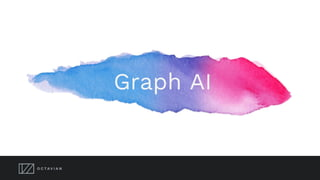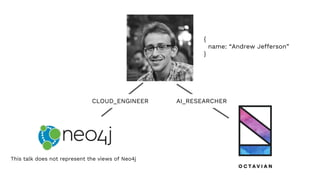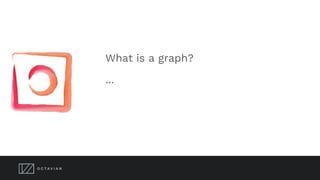Successfully reported this slideshow.

# Knowledge graphs, meet Deep Learning×

# Knowledge graphs, meet Deep Learning

Knowledge graphs generation is outpacing the ability to intelligently use the information that they contain. Octavian's work is pioneering Graph Artificial Intelligence to provide the brains to make knowledge graphs useful.

Our neural networks can take questions and knowledge graphs and return answers. Imagine:

Taking a neural network approach is important because neural networks deal better with the noise in data and variety in schema. Using neural networks allows people to ask questions of the knowledge graph in their own words, not via code or query languages.

Octavian's approach is to develop neural networks that can learn to manipulate graph knowledge into answers. This approach is radically different to using networks to generate graph embeddings. We believe this approach could transform how we interact with databases.

Knowledge graphs generation is outpacing the ability to intelligently use the information that they contain. Octavian's work is pioneering Graph Artificial Intelligence to provide the brains to make knowledge graphs useful.

Our neural networks can take questions and knowledge graphs and return answers. Imagine:

Taking a neural network approach is important because neural networks deal better with the noise in data and variety in schema. Using neural networks allows people to ask questions of the knowledge graph in their own words, not via code or query languages.

Octavian's approach is to develop neural networks that can learn to manipulate graph knowledge into answers. This approach is radically different to using networks to generate graph embeddings. We believe this approach could transform how we interact with databases.

### Knowledge graphs, meet Deep Learning

1. 1. Graph AI
2. 2. CLOUD_ENGINEER AI_RESEARCHER This talk does not represent the views of Neo4j { name: “Andrew Jefferson” }
3. 3. What is a graph? ...
4. 4. What is a graph? Why are we interested in graphs? ...
5. 5. (Graphs)-[ARE]->(Everywhere)
6. 6. What is a graph? Why are we interested in graphs? What is AI? ...
7. 7. What is a graph? Why are we interested in graphs? What is AI? What is Deep Learning? ...
8. 8. 90% accuracy
9. 9. Machine learning trained_model = train(model, data) learned_weights = trained_model.get_weights() prediction = trained_model.predict(instance)
10. 10. Machine learning trained_model = train(model, data) learned_weights = trained_model.get_parameters() prediction = trained_model.predict(instance) Model Learned Params Training Data Linear Regression y = mx + c m, c List<(x, y)> Basic Classifier P(class | x) = softmax((dense(w, x)) w class set List<(x, class)> NN Embedding P(context | word) = NN(word) word embedding, NN weights word dictionary List<(context, word)>
11. 11. Deep Learning = ML using Deep Neural Networks trained using gradient descent
12. 12. What is a graph? Why are we interested in graphs? What is Deep Learning? What is Deep Learning on graphs? ...
13. 13. Model Learned Params Data Linear Regression y = mx + c m List<(x, y)> Image Classifier P(class | image) = CNN(image) CNN weights List<(image, class)> NN Embedding P(path | node) = NN(path) node embedding, NN weights Node dictionary List<(path, node)> Graph Regression node.prop = F([sub]graph) F weights List<(node, [sub]graph)> or Graph, pattern Graph Classifier P(class | [sub]graph) = F([sub]graph) F weights List<([sub]graph, class)> or Graph, pattern Graph Embedder P(subgraph | node) = F(graph) node embedding, F weights Graph
14. 14. How’s that going?
15. 15. How to DL Graphs - the dimensionality challenge! How to encode a variable graph into a fixed size matrix?
16. 16. Graphs, Neural Networks and Structural Priors “DL is good for unstructured data” ... bad at structured data: THIS IS NOT TRUE DL is good at very specific data structures: Images: Sequences:
17. 17. Images: Sequences:
18. 18. Images: Convolutional Network
19. 19. What about graphs? Graph AI is more than a “how to vectorise a graph” challenge!
20. 20. What about graphs? Graph AI is more than a “how to vectorise a graph” challenge!
21. 21. What about graphs? Graph AI is more than a “how to vectorise a graph” challenge!
22. 22. How to do Deep Learning on Graphs Graphs - the dimensionality challenge How to encode a variable graph into a fixed size matrix? Graphs - the inductive bias challenge? How can we structure a neural network to retain graph structural priors?
23. 23. Graph Networks
24. 24. Graph Memory Network
25. 25. What is a graph? Why are we interested in graphs? What is Deep Learning? What is Deep Learning on graphs? Does it work?
26. 26. CLEVR-GRAPH https://github.com/Octavian-ai/clevr-graph A synthetic dataset where 100% accuracy is achievable.
27. 27. CLEVR-GRAPH https://github.com/Octavian-ai/clevr-graph
28. 28. CLEVR-GRAPH https://github.com/Octavian-ai/clevr-graph
29. 29. What is the cleanliness level of {Station} station? 99.9% accuracy after 10k training steps How big is {Station}? What music plays at {Station}? What architectural style is {Station}? Describe {Station} station's architectural style. Is there disabled access at {Station}? Does {Station} have rail connections? Can you get rail connections at {Station}? Are {Station} and {Station} adjacent? 99% accuracy after 20k training steps Which {Architecture} station is adjacent to {Station}? 98.8% accuracy after 30k training steps How many stations are between {Station} and {Station}? 98% accuracy up to ~9 apart after 25k training steps Are {Station} and {Station} connected by the same station? 98% accuracy after 20k steps Which station is adjacent to {Station} and {Station}? Is there a station called {Station}? 99.9% accuracy after 30k training steps Is there a station called {FakeStationName}? Are {Station} and {Station} on the same line? Not yet tested How many architectural styles does {Line} pass through? Not yet tested How many music styles does {Line} pass through? Not yet tested How many sizes of station does {Line} pass through? Not yet tested How many stations with rail connections does {Line} pass through? Not yet tested Which lines is {Station} on? Not yet tested How many lines is {Station} on? Not yet tested Which stations does {Line} pass through? Not yet tested
30. 30. What is a graph? Why are we interested in graphs? What is Deep Learning? What is Deep Learning on graphs? ?Does it work? … How does it work?
31. 31. Graph Q&A “How many stations are between Bank and Temple?” Graph Networks gave us a method for propagating information through the graph. How do we prime the graph to answer our specific query?
32. 32. How to do Deep Learning on Graphs Graphs - the dimensionality challenge How to encode a variable graph into a fixed size matrix? Graphs - the inductive bias challenge? How can we structure a neural network to retain graph structural priors? Graphs - the starting condition challenge? How do we prime the graph to answer our specific query?
33. 33. Attention
34. 34. https://github.com/Octavian-ai/mac-graph
35. 35. Deep Learning on Graphs ● DL model that can learn how to navigate a graph schema ● End-to-end training on List<(x, y, graph)> ● A single DL model can learn to solve multiple problems ○ Using multiple algorithms
36. 36. Thanks https://octavian.ai @Octavian_ai @EastlondonDev hello@octavian.ai
37. 37. Regular vs Graph Classifier Model Learned Weights Data P(class | x_features) = NN(x_features) NN weights List<(x_features, class)> Works well if your features are easily vectorised and uniform length. No vectorisation required, can handle extremely heterogeneous data. Model Learned Weights Data P(class | [sub]graph) = NN([sub]graph) NN weights List<([sub]graph, class)>
38. 38. Images: Sequences: Retail: London Underground:
39. 39. We can barely handle the small data sets intelligently, let alone the large ones Issues - Absence of useful graph techniques - Either large scale and statistical - Rule based and inflexible Neither help deal with real world graph queries:
40. 40. Trusted consumer brand Quality handheld electronics manufacturing Battery and HDD technology (from laptops) Integration with music software on a pc Fast data transfer (Firewire)
41. 41. Battery and HDD technology (from laptops) Integration with music software on a pc Fast data transfer (Firewire) 1995 Trusted consumer brand Quality handheld electronics manufacturing
42. 42. Why are graphs useful?
43. 43. Graph Analytics Pattern matching Recommendations Fraud Detection Optimisation
44. 44. What am I going to tell you? 1) What is a graph and why are we interested in graphs? ✓ 2) a tiny nod to "traditional" graph ML 3) Terminology: DL := "Deep learning with Neural Networks" (optionally Reinforcement) 4) Deep Learning is cool, some examples 5) People think DL is good for unstructured data and bad at structured data: THIS IS NOT TRUE - DL is good at very specific data structures: grids and sequences of single entities 6) General state of the world of "AI" on graphs (basically a walkthrough of the Relational Inductuve Bias' paper) 7) Some specific knowledge graph architecture
45. 45. Many kinds of graphs, Many kinds of AI Connectivity Graph Hallmarks: Concerned with individual entities. Has very small number of node and relationship types (often just 1 of each). Distance between nodes is meaningful. Generally a single connected graph Example: Simple social graph, Netflix graph, Transit graph Algorithms: Shortest path, Neighbourhood, Recommendation, Link Prediction Knowledge Graph Hallmarks: Describes an abstraction. Has large number of node _or_ relationship types (or both). Distance between nodes is not meaningful. May be multiple unconnected graphs Example: Medical research database, Marketing graph, Ontologies Algorithms: Inference, Question answering, Property Prediction
46. 46. Many kinds of graphs, Many kinds of AI Hybrid Graphs Hallmarks: Distance between nodes may or may not be meaningful depending on the relationship type. Example: Online shop database, Communication graph, Sophisticated Transaction graph Algorithms: Inference, Recommendation, Link/Property Prediction We can classify graphs in all kinds of ways, but it’s a relatively futile exercise Graph AI may be quite specific, predicated on a particular graph properties, or it might be flexible. We are interested in both!
47. 47. Graph Classifier Train( model, (y)-[..2]-(), y.is_fraud ) Train( model, (p:Person)<-[r:FRIEND_OF]-(:Person)->(), p.favorite_animal ) Train( model, (c:Company)-[..3]->(), c.future_value )
48. 48. How to DL? I will show four different examples. Each example has a different Deep Learning inspired method to solve a different kind of problem
49. 49. How to DL? Learn a node or edge embedding using a mathematical model Does not necessarily involve Neural Networks! We have to invent/choose the mathematical function we will use Overcomes the dimensionality challenge using Random Walks Example: Review prediction with dot https://medium.com/octavian-ai/review-prediction-with-neo4j-and-tensorflow-1c d33996632a DeepWalk, node2vec create Word2Vec style embeddings based on _connections_ DeepGL creates features based on other graph properties
50. 50. How to DL? Learn an embedding _and_ an interaction fn at the same time Example? Deep
51. 51. How to DL? Construct graph queries using DL (Tool Use) Translate questions into graph queries: https://medium.com/octavian-ai/answer ing-english-questions-using-knowledge -graphs-and-sequence-translation-2ac baa35a21d Sidesteps the dimensionality challenge completely
52. 52. How to DL? Treat nodes and relations as tables Example: property queries on CLEVR graph: https://medium.com/octavian-ai/graphs-and-neural-networks-reading-node-propert ies-2c91625980eb Uses attention to overcome the dimensionality challenge. Doesn’t use the graph structure though
53. 53. How to DL? Transmit signals from nodes in the graph Uses attention and message passing
54. 54. Knowledge Graphs Connections (relations) are important Enormous linked data sets exist (knowledge graphs) Small linked data sets also exist (legal cases, personal graphs)
55. 55. Existing Graph DL 1) Categorisation 2) Link Prediction 3) Chemistry These don’t help much with our knowledge graphs! We want something different to existing graph DL 1) Should work on relatively small graphs 2) Should facilitate querying the graph to extract information/predictions based on the graph and the query
56. 56. Deep Learning for Knowledge Graphs Step 1: We need a metric that we can use to test our “AI”. Step 2:
57. 57. Deep Learning for Knowledge Graphs Node Properties and Graph Structure (relationships) are both important to a knowledge graph. Knowledge graph queries depend on multiple nodes & graph structure. Node/edge embeddings don’t capture the necessary information.
58. 58. Everything is a graph ● Organisation Chart ● Social Network ● Product Reviews ● Blockchain ● Supply Chain ● Chemical Structure ● Mechanical Structure ● Travel Itinerary ● The Internet ●